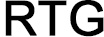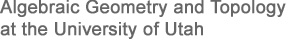# Introduction to Algebraic Geometry

## May 16-27, 2016

Instructor: Christopher Hacon

WEB L120, University of Utah

Algebraic Geometry is, at its core, the study of the solution sets to polynomial equations. Its roots date back to the ancient Greeks and the subject closely related to many different fields in mathematics and beyond (such as algebra, differential geometry, topology, analysis, number theory and mathematical physics to name a few). In this course we will give an introduction to Algebraic Geometry which is appropriate for the advanced undergraduate student which emphasizes the theoretical foundations as well as concrete computations and examples. We will also explore additional topics related to modern research.

The topics covered in this course will include: Algebraic sets and varieties, Zariski topology, the Nullstellensatz, projective spaces and varieties, Bezout's Theorem, rational functions and rational maps. dimension theory, singular and non-singular varieties, tangent and cotangent spaces. the Bertini-Sard Theorem. We will also discuss Groebner basis and perform many explicit computer aided computations.

Prerequisites: Linear Algebra, Undergraduate Algebra (group theory and ring theory) and basic knowledge of set theoretic topology

References:
1. "An Invitation to Algebraic Geometry" Smith Kahanpaa, Kekalainen and Traves, Universitext Springer-Verlag, New York, 2000. xii+155 pp. ISBN: 0-387-98980-3
2. "Ideals, varieties, and algorithms. An introduction to computational algebraic geometry and commutative algebra." Undergraduate Texts in Mathematics, Springer Verlag, New York, New York, 1992. xii+513 pp. ISBN: 0-387-97847-X

Financial support is available to US citizens and permanent residents and all eligible students who are accepted will have all of their costs associated with attendance covered.

Application is now closed

Course Poster